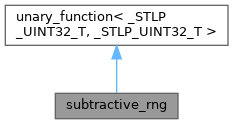ReactOS 0.4.15-dev-5865-g640e228
subtractive_rng Class Reference

`#include <_function.h>`

Inheritance diagram for subtractive_rng:[legend]
Collaboration diagram for subtractive_rng:[legend]

## Public Member Functions

_STLP_UINT32_T operator() (_STLP_UINT32_T __limit)

void _M_initialize (_STLP_UINT32_T __seed)

subtractive_rng (unsigned int __seed)

subtractive_rng ()

## Private Attributes

_STLP_UINT32_T _M_table 

_STLP_UINT32_T _M_index1

_STLP_UINT32_T _M_index2Public Types inherited from unary_function< _STLP_UINT32_T, _STLP_UINT32_T >
typedef _STLP_UINT32_T argument_type

typedef _STLP_UINT32_T result_typeProtected Member Functions inherited from unary_function< _STLP_UINT32_T, _STLP_UINT32_T >
~unary_function ()

## Detailed Description

Definition at line 388 of file _function.h.

## ◆ subtractive_rng() [1/2]

 subtractive_rng::subtractive_rng ( unsigned int __seed )
inline

Definition at line 419 of file _function.h.

419{ _M_initialize(__seed); }
void _M_initialize(_STLP_UINT32_T __seed)
Definition: _function.h:401

## ◆ subtractive_rng() [2/2]

 subtractive_rng::subtractive_rng ( )
inline

Definition at line 420 of file _function.h.

420{ _M_initialize(161803398ul); }

## ◆ _M_initialize()

 void subtractive_rng::_M_initialize ( _STLP_UINT32_T __seed )
inline

Definition at line 401 of file _function.h.

401 {
402 _STLP_UINT32_T __k = 1;
403 _M_table = __seed;
404 _STLP_UINT32_T __i;
405 for (__i = 0; __i < 54; __i++) {
406 _STLP_UINT32_T __ii = (21 * (__i + 1) % 55) - 1;
407 _M_table[__ii] = __k;
408 __k = __seed - __k;
409 __seed = _M_table[__ii];
410 }
411 for (int __loop = 0; __loop < 4; __loop++) {
412 for (__i = 0; __i < 55; __i++)
413 _M_table[__i] = _M_table[__i] - _M_table[(1 + __i + 30) % 55];
414 }
415 _M_index1 = 0;
416 _M_index2 = 31;
417 }
#define _STLP_UINT32_T
Definition: _apple.h:37
_STLP_UINT32_T _M_index2
Definition: _function.h:392
_STLP_UINT32_T _M_index1
Definition: _function.h:391
_STLP_UINT32_T _M_table
Definition: _function.h:390

Referenced by subtractive_rng().

## ◆ operator()()

 _STLP_UINT32_T subtractive_rng::operator() ( _STLP_UINT32_T __limit )
inline

Definition at line 394 of file _function.h.

394 {
395 _M_index1 = (_M_index1 + 1) % 55;
396 _M_index2 = (_M_index2 + 1) % 55;
398 return _M_table[_M_index1] % __limit;
399 }

## ◆ _M_index1

 _STLP_UINT32_T subtractive_rng::_M_index1
private

Definition at line 391 of file _function.h.

Referenced by _M_initialize(), and operator()().

## ◆ _M_index2

 _STLP_UINT32_T subtractive_rng::_M_index2
private

Definition at line 392 of file _function.h.

Referenced by _M_initialize(), and operator()().

## ◆ _M_table

 _STLP_UINT32_T subtractive_rng::_M_table
private

Definition at line 390 of file _function.h.

Referenced by _M_initialize(), and operator()().

The documentation for this class was generated from the following file: